# Fractions

This example teaches you how to enter a fraction in Excel and how to change the format of a fraction.

1. To enter the mixed fraction 1 4/7 (one and four sevenths), type 1 4/7. To enter the fraction 4/7 (four sevenths) into cell A1, select cell A1 and type 0 4/7 (with a 0 or Excel thinks you want to enter a date).Now that you've entered a fraction correctly, Excel has applied a Fraction format to cell A1 and you can edit the fraction by simply typing 5/7 (without a 0). You can find the decimal value of the fraction in the formula bar.

2. Let's take a look at the applied Fraction format. Select cell A1, right click, and then click Format Cells.

Excel has applied the Up to one digit type.Note: to enter a fraction like 4/11 into cell A1, change the type to Up to two digits. To enter a fraction like 1/148 into cell A1, change the type to Up to three digits.

3a. Excel rounds your fraction if it cannot display the right fraction. For example, if you type 4/11 into cell A1 (with the Up to one digit type applied), Excel displays 1/3. The formula bar always shows the correct decimal value.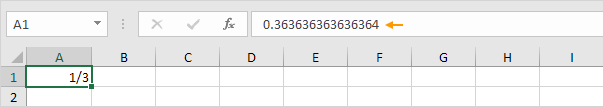3b. Change the type to Up to two digits.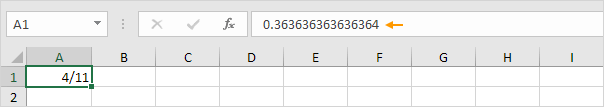Note: of course, if you type 0 4/11 into a new cell, Excel automatically applies the Up to two digits type and correctly displays 4/11.

4. Excel always reduces a fraction to its smallest denominator (bottom number of the fraction). If you enter the fraction 2/8, Excel converts the fraction to 1/4. However, you can use the As eights (4/8) type to fix the denominator to 8.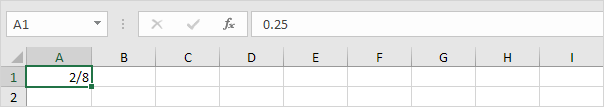5. Sometimes, you cannot find the right denominator for your fraction. For example, you want to display 40/50, but Excel converts 40/50 to 4/5. However, the As fiftieths (25/50) type is not available. Create a custom number format (# ??/50) to fix this.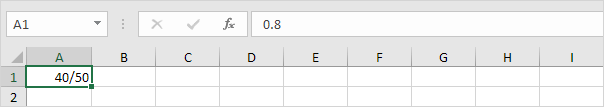Note: change the 50 to 60 to create the As sixtieths (30/60) type, etc.

If you don't want to use a fraction in a calculation, but only want to diplay a fraction somewhere on your worksheet, read on.

6. On the Insert tab, in the Symbols group, click the Pi symbol to add a new equation.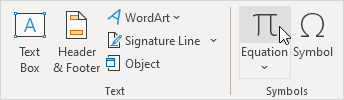7. Add a fraction to the equation.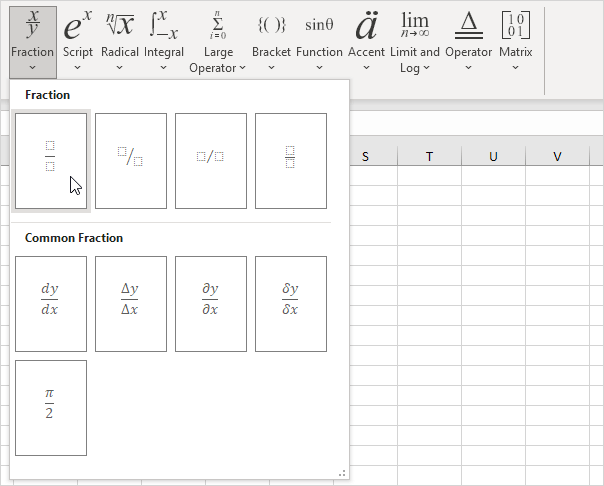8. Add a numerator (top number of the fraction) and a denominator (bottom number of the fraction).

Result: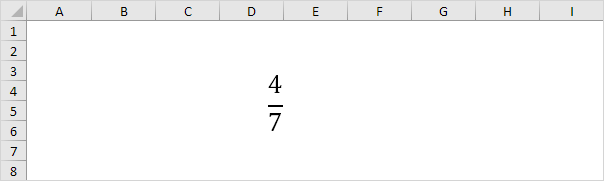Note: we increased the font size of the fraction and changed the background color of the cells to White.

Go to Next Chapter: Find & Select